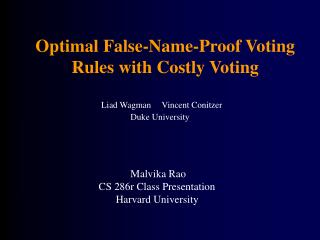DownloadDownload PresentationOptimal False-Name-Proof Voting Rules with Costly Voting

# Optimal False-Name-Proof Voting Rules with Costly Voting

Download Presentation## Optimal False-Name-Proof Voting Rules with Costly Voting

- - - - - - - - - - - - - - - - - - - - - - - - - - - E N D - - - - - - - - - - - - - - - - - - - - - - - - - - -
##### Presentation Transcript

1. Optimal False-Name-Proof Voting Rules with Costly Voting Liad Wagman Vincent Conitzer Duke University Malvika Rao CS 286r Class Presentation Harvard University

2. Overview • Introduction • Definitions • False-name-proof voting rule for 2 alternatives • Group false-name-proofness • False-name-proof voting rule for 3 alternatives • Discussion

3. Introduction • Introducing costs… • Previous rules without costs unresponsive to agent preferences. • Idea: no one ever benefits by voting additional times. • Because we now have costs we are tying utility to money. So people’s utility function becomes comparable.

4. Definition 1 (State): A state consists of a pair (xA, xB), where xj ≥ 0 is the # of votes for j in {A, B}. Definition 2 (Voting Rule): A voting rule is a mapping from the set of states to the set of probability distributions over outcomes. The probability that alternative j in {A, B} is selected in state (xA, xB) is denoted by Pj(xA, xB). Definition 3 (Neutrality): A voting rule is neutral if PA(x, y) = PB(y, x) . Definitions (2 alternatives)

5. Let tiA and tiB be the # of times agent i votes for A and B. If i prefers alternative j then i’s expected utility ui(xA, xB, tiA, tiB) = Pj(xA + tiA, xB + tiB) - (tiA + tiB - 1)c. Definition 4 (Voluntary Participation): A voting rule satisfies voluntary participation if for an agent i who prefers A, for all (xA, xB), ui(xA, xB, 1, 0) ≥ ui(xA, xB, 0, 0) . Definition 5 (Strategy-proofness): A voting rule is strategy-proof if for an agent i who prefers A, for all (xA, xB), ui(xA, xB, 1, 0) ≥ ui(xA, xB, 0, 1) . Definitions (2 alternatives)

6. Definition 6 (False-name-proofness): A voting rule is false-name-proof (with costs) if for an agent i who prefers A, for all (xA, xB), for all tiA ≥ 1 and tiB, ui(xA, xB, 1, 0) ≥ ui(xA, xB, tiA, tiB) . Definition 7 (Strong optimality): A neutral false-name-proof voting rule P that satisfies voluntary participation is strongly optimal if for any other such rule P´, for any state (xA, xB) where xA ≥ xB, we have PA(xA, xB) ≥ P´A(xA, xB). Definitions (2 alternatives)

7. FNP2: Suppose xA ≥ xB. Then PA(xA, xB) = 1 if xA > xB = 0, PA(xA, xB) = min{1, 1/2 + c(xA - xB)} if xA ≥ xB > 0 or xA = xB = 0. Theorem: FNP2 is the unique strongly optimal neutral false-name-proof voting rule with 2 alternatives that satisfies voluntary participation. False-name-proof voting rule for 2 alternatives

8. Proof: FNP2 is strongly optimal By neutrality for any x ≥ 0 P´A(x, x) = 1/2. By false-name-proofness for any x > 0 P´A(x+1, x) - P´A(x, x) ≤ c. So P´A(x+1, x) ≤ 1/2 + c. Similarly P´A(x+2, x) ≤ P´A(x+1, x) + c ≤ 1/2 + 2c. For any t > 0 P´A(x+t,x) ≤ 1/2 + tc. Since P´A(x+t,x) ≤ 1, P´A(x+t,x) ≤ min{1, 1/2 + tc}. But PA(x+t,x) = min{1, 1/2 + tc}. False-name-proof voting rule for 2 alternatives

9. FNP2 Responsiveness • Example: c = 0.15.

10. FNP2 Responsiveness • Convergence to majority winner as n --> ∞.

11. FNP2 Responsiveness • Average probability that FNP2 and majority rule disagree as a function of c.

12. FNP2 Responsiveness • Average probability that FNP2 and majority rule disagree as a function of p (probability agent prefers A).

13. FNP2 is not group false-name-proof. Consider the example: c = 0.15, xA = xB = 2. If the 2 agents that prefer A each cast an additional vote then A now wins with probability 0.8. Each agent is 0.3 - 0.15 = 0.15 better off. A rule is group false-name-proof (with costs and transfers) if for all k ≥ 1, for all (xA, xB), for all tA ≥ k and tB, PA(xA + k, xB) ≥ PA(xA + tA, xB + tB) - c(tA + tB - k)/k . Group false-name-proof voting rule for 2 alternatives

14. Strongly optimal GFNP2: Suppose xA ≥ xB. Then PA(xA, xB) = 1 if xA > xB = 0, PA(xA, xB) = 1/2 if xA = xB = 0, PA(xA, xB) = min{1, 1/2 + ∑k (c/k) for k = xBto xA-1} if xA ≥ xB > 0. As n --> ∞ GFNP2 yields the opposite result from the majority rule at least 40% of the time. There is no finite c such that GFNP2 coincides with the majority rule. Group false-name-proof voting rule for 2 alternatives

15. Strong optimality: Voting rule P is strongly optimal if for any other rule P´, for any (xA, xB , xC) where xA ≥ xB ≥ xC ≥ 1, either PA (xA, xB , xC) > P´A (xA, xB , xC); or PA (xA, xB , xC) = P´A (xA, xB , xC) and PB (xA, xB , xC) ≥ P´B (xA, xB , xC) . FNP3: Suppose xA ≥ xB ≥ xC ≥ 1. Then PA (xA, xB , xC) = min{1, 1/2 + c(xA - xB) - 1/2 max{0, 1/3 - c(xB - xC)}} PC (xA, xB , xC) = max{0, 1/3 - c((xA + xB)/2 - xC)} PB (xA, xB , xC) = 1 - PA (xA, xB , xC) - PC (xA, xB , xC) False-name-proof voting rule for 3 alternatives

16. Discussion • 4+ alternatives… • How can we improve group false-name-proofness? • GFNP3? • Continuous preferences • Bayes-Nash